Following are answer explanations to the problems located throughout the previous Chemistry articles. Hopefully, you've challenged yourself to attempt some of these as you've reviewed the various topics. If not, you can use these problems as additional review now.

1. By mass, the compound is 26.19% nitrogen, 7.55% hydrogen, and 66.26% chlorine. (Total mass:53.         50 g)2. The simplest formula is K 2CuF 4.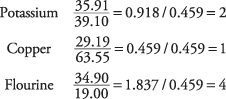3. There are 6.37 moles of C 6H 5Br.4. The neon has a mass of 4.5 grams.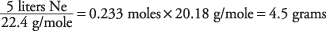5. The reaction uses 2.79 liters of oxygen.

1 mole CH 4 = 1(12.01) + 4(1.01) = 16.05 grams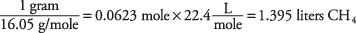The reaction coefficients denote the relative volumes, so the volume of O 2 is twice that of CH 4 = 2 ×        1.395 L = 2.79 liters.

6. Nuclei B and C are isotopes of magnesium, the element with atomic number 12. Nuclei A and B both           have a mass of approximately 24 atomic mass units because their nucleons sum to 24.

7. Natural silver is 48.15% silver‐109.8. The nucleus is radium‐226, also written. The atomic mass decreased by four, the mass of the       alpha particle. The atomic number decreased by two because the alpha particle carried off two protons. The problem asks for the mass number “226,” the atomic number “88,” and the name of the element “radium.”

9. Aluminum has three valence electrons; whereas oxygen has six. Remember that you count columns from the left margin of the periodic table.

10. The Lewis diagram for H 2S is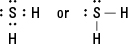11. The electronegativity difference of magnesium and chlorine is 1.8,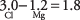which corresponds to a bond with 52% ionic character and 48% covalent character. Such an  intermediate bond is called polar.

12. The three isomers of C 5H 12 are shown in the following example. The essential feature is the bonding of carbons. In the first molecule, no carbon is bonded to more than two carbons, the second molecule has a carbon bonded to three carbons, and the third molecule has a carbon bonded to four carbons.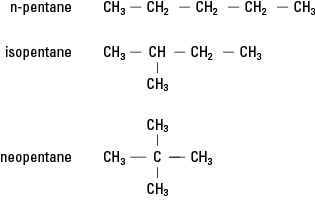13. The addition of hydrogen converts acetylene to ethane: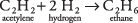Because the number of moles of hydrogen is twice that of acetylene, the reaction requires 200 liters of hydrogen, double that of acetylene.

14. It is an aldehyde with the structural formula:15. The minimum pressure for liquid CO 2 is 5.1 atmospheres.

16. At –64°C, the solid CO 2 sublimates to the gas state.

17. The total heat needed is 49,831 joules.18. The pressure equals 0.804 atmosphere.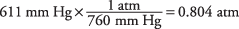19. The required pressure is 1.654 atmospheres.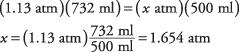20. The chilled temperature is –217.63°C.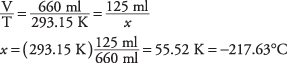21. There are 1.5 × 10 24 hydrogen atoms.22. The carbon monoxide occupies 28,499 liters.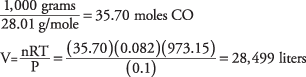23. The ozone molecule has the formula O 3.24. The solution is 0.592 in glucose.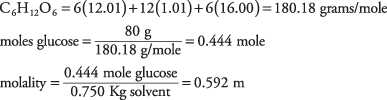25. The solution is 0.36 mole fraction alcohol.

CH 3OH = 32.05 grams/mole H 2O = 18.02 grams/mole moles alcohol = 100 g/32.05 g/mole =   3.12 mole moles water = 100 g/18.02 g/mole = 5.55 mole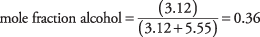26. The amount of CuCl is 0.00152 mole. If the powder dissolved completely, the solution would be   0.00152 molar with respect to both Cu + and Cl .

[Cu +] [Cl ] = (0.00152) 2 = 2.3 × 10 –6

Because that product exceeds the solubility product given in the chart as 1.1 × 10 ‐6, which is the   value for a saturated solution, the powder will not completely dissolve.

27. The solubility of aluminum hydroxide is 0.00843 gram per liter. The Al(OH) 3 dissociates to 4 ions   with the concentration of OH being three times that of A 3+.28. The sodium chloride solution boils at 100.87°C.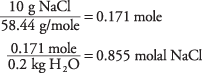Each formula unit yields 2 ions; so, the total molality of the ions is twice that, or 1.712 m. The change in boiling point is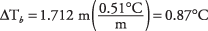and that value is added to the 100° boiling point of pure water.

29. The molecular weight of brucine is approximately 394. Table states that pure chloroform freezes at –   63.5°C.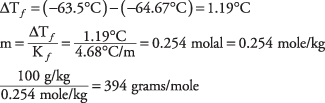30. The solution is alkaline with pH = 8.34.31. The solution required 0.056 mole of acetic acid.

From the pH, [H +] = 10 –3 and [CH 3COO ] must be the same.

32. The conjugate base ofis the carbonate ion, formed by the loss of a proton. The   conjugate acid is carbonic acid H 2CO 3, formed as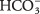gains a proton.

33.34. Nitrogen has the oxidation number –3 in Mg 3N 2 and +5 in HNO 3. For Mg 3N 2For HNO 3,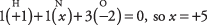Notice that the oxidation number per atom is multiplied by the number of atoms in the formula unit.

35. Carbon is oxidized and iodine is reduced, so CO is the reducing agent and I 2O 5 is the oxidizing   agent.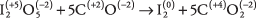Each of the five carbon atoms loses two electrons, and each of the two iodine atoms gains five     electrons.

36. Only manganese and oxygen have variable oxidation numbers.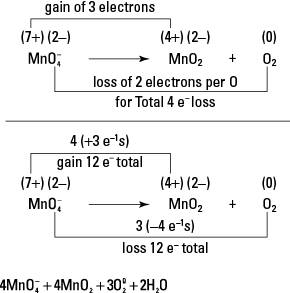37. The silver is deposited from solution as the iron dissolves.38. The lithium‐fluorine battery yields 5.91 volts.39. The electrolysis requires 111.2 faradays of electricity.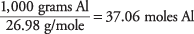Al (+3) + 3e → Al (0) (reduction) moles of electrons = 3 × moles Al = 3 × 37.06 = 111.2 moles e

40. The initial reaction is:Conclusion: BrCl will decompose to form Br 2 and Cl 2 to restore the equilibrium.

41. The value of PSO 3 is 0.274 atmosphere.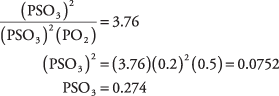42. The mass of N 2O 4 would increase and NO 2 would decrease. The volume coefficient of the left side   (1) is less than that of the right side (2), so a conversion of NO 2 to N 2O 4 would minimize the   increase in pressure.

43. The mass of NO 2 would increase, and N 2O 4 would decrease. Because the forward reaction is   endothermic,the conversion of N 2O 4 to NO 2 would absorb heat and minimize the increase in temperature.

44. The enthalpy of reaction is +33.7 kcal, so the reaction is endothermic.45. The exothermic reaction releases 27.8 kilocalories of heat.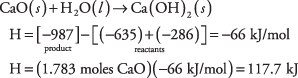46. The free energy change is 48.3 kcal; because this is positive, the reaction would not proceed.47. The temperature change reverses the reaction direction. From the standard values that are given,   you can calculate that

ΔH = 58.02 kJ

ΔS = 176.7 J/deg = 0.1767 kJ/deg

and then substitute these into

ΔG = ΔH – TΔS

At 25°C = 298 K, the free energy favors N 2O 4:

ΔG = (58.02 kJ) – (298)(0.1767 kJ/deg) = 5.362 kJ

At 100°C = 373 K, the free energy favors NO 2:

ΔG = (58.02 kJ) – (373)(0.1767 kJ/deg) = –1.886 kJ